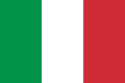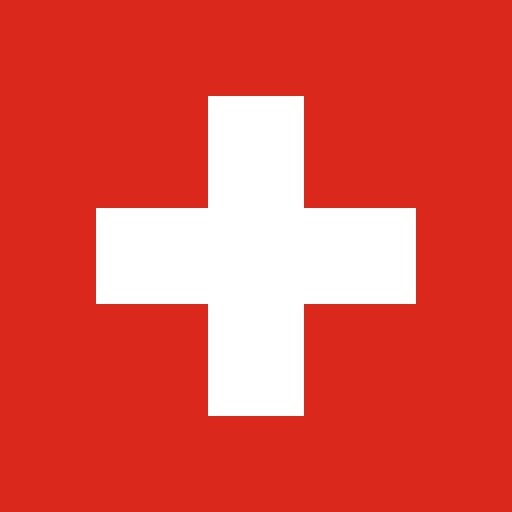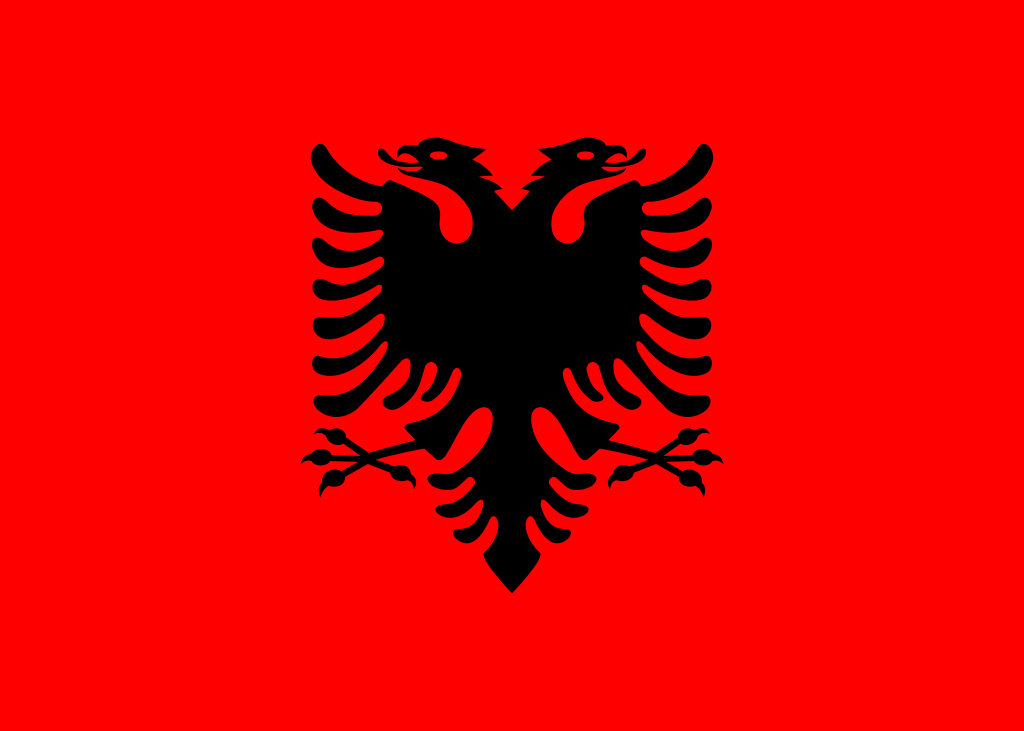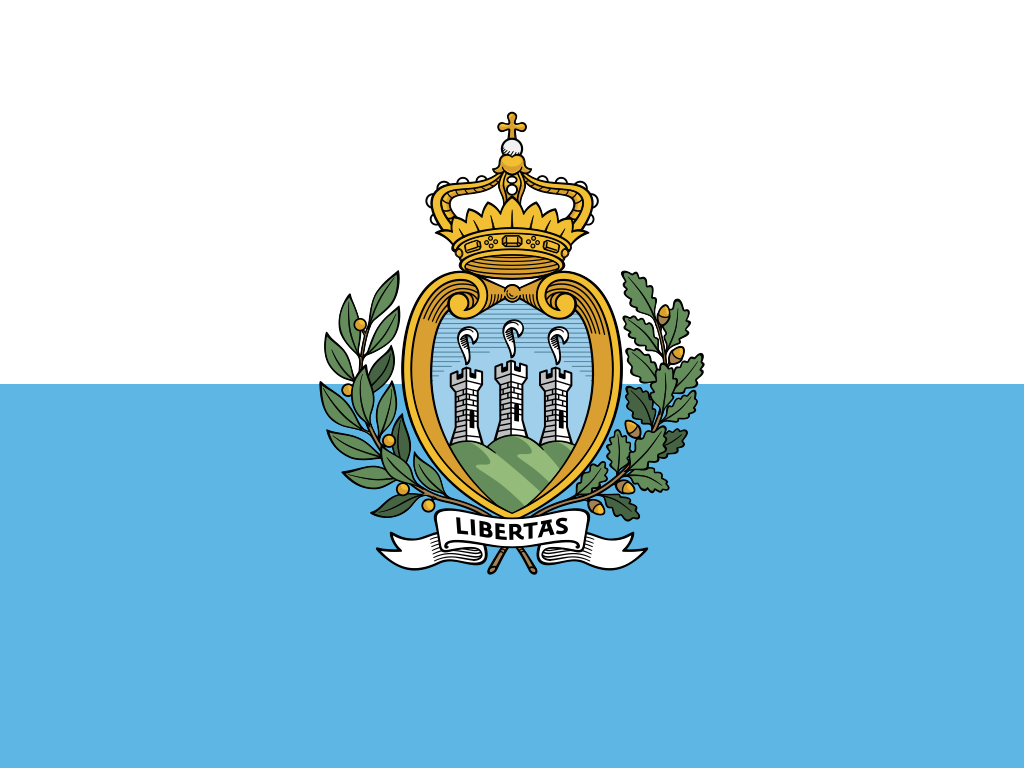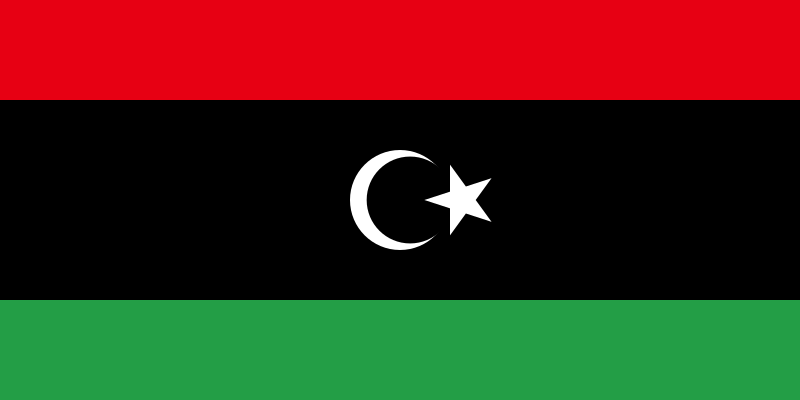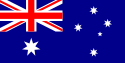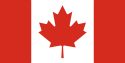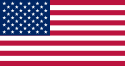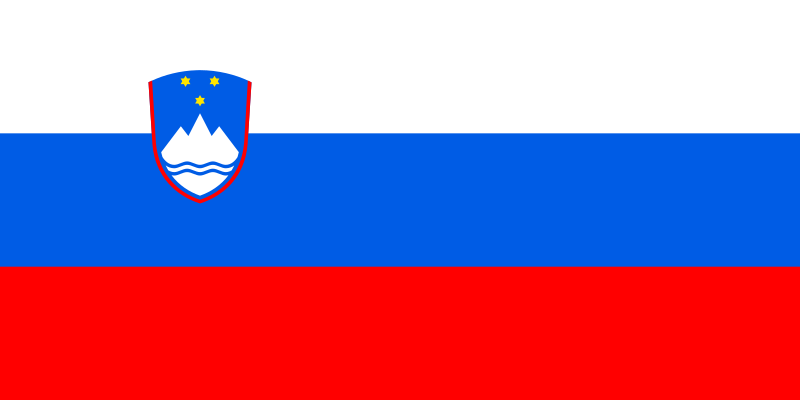意大利语字母 A a (À) (à) B b C c D d E e (È) (è) (É) (é) F f G g H h I i (Ì) (ì) (Í) (í) (Î) (î) J j K k L l M m N n O o (Ò) (ò) (Ó) (ó) P p Q q R r S s T t U u (Ù) (ù) (Ú) (ú) V v W w X x Y y Z z